Disclosure: This post may contain affiliate links, meaning when you click the links and make a purchase, we receive a commission.

# Financial Planning with Excel Solver [2 Case Studies]

Most of us need to determine the monthly payment or the amount of money to be saved for retirement over life. It is not easy to do such kind of calculations manually. Fortunately, Excel Solver can also be applied in similar situations. As with other articles, I suggest you read my previous post – Using Excel Solver to Determine the Optimal Product Mixtures – if you are not familiar with Excel Solver.

## Case 1: Calculate monthly payment

Suppose that you are borrowing \$15,000 to buy a new car and plan to make 10 end-of-month payments. The annual interest rate on the loan is 10 percent. The car dealer allows you to make the monthly payment for Month 31-60 equal to half the payments for Months 1 through 60. Assume that your payment occurs at the end of each month, what is the payment during each month?

Read More: GRG Multistart and Evolutionary Excel Solver Engines [2 Case Studies]

Figure 1.1 shows how to set the model for this problem. Beginning balance was put in column C and cell C3 equal to \$15,000 which is the total amount of money that you borrowed. Monthly payment is what you seek and D3 is our target cell. The interesting thing is that Monthly payment is also by changing cell and thus I left it blank at the moment.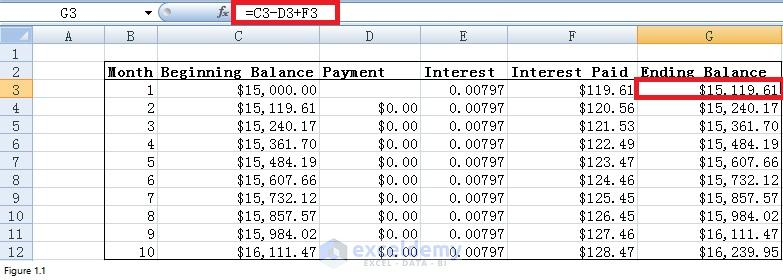Figure 1.1

As for column E, we should fill into monthly interest rate instead of annual interest rate. Therefore, I had to do a conversion. I did a conversion on this web page – http://www.stoozing.com/calculator/apr-rate-converter.phpv. Figure 1.2 shows the returned monthly interest rate is 0.7974 and I put 0.00797 in column E. Because the payment occurs at the end of each month, I put formula “=C3*E3” in cell F3 to compute interest incurred on the balance at the beginning of the first month. By copying this formula into cells F4 through F12, the interest that you have to pay for other months can be calculated. The relationship between column C, column D, column E, column F and column G can be described by the following equation:

(Unpaid loan balance at end of period t) = (Unpaid loan balance at beginning of period t) – [(Month t payment) – (Month t interest paid)]

Formula “=C3-D3+F3” which is derived from this equation was put in cell G3 to compute the ending balance in the first year. Copy this formula into range G4:G12 to calculate ending balance for other months. We all know that ending balance of this month will be the beginning balance of next month. Therefore, formula “=G3” was entered into cell C4 and copied into range C5:C12 to display the beginning balance for months 3 to month 10.

Read More: Excel Solver with a Case Study [Rate Your Sports Team]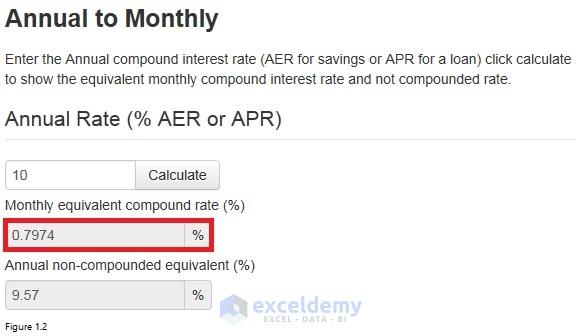Figure 1.2

Click on Solver in Analysis group to open Solver Parameters dialog box. The solver Parameter dialog box for this model is shown in Figure 1.3. The constraint for this model is that the ending balance for month 10 should be \$0.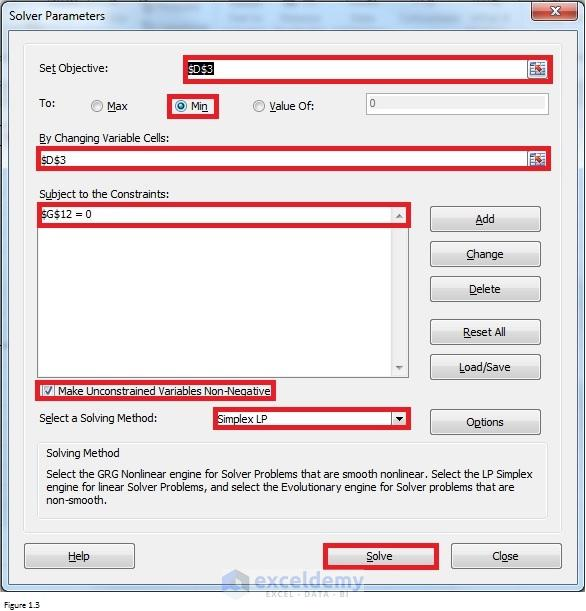Figure 1.3

After clicking Solve in the Solver Parameters dialog box, you can find that your monthly payment for the first 5 months is \$2,075.03. And during other 5 months, you have to pay \$1,037.51.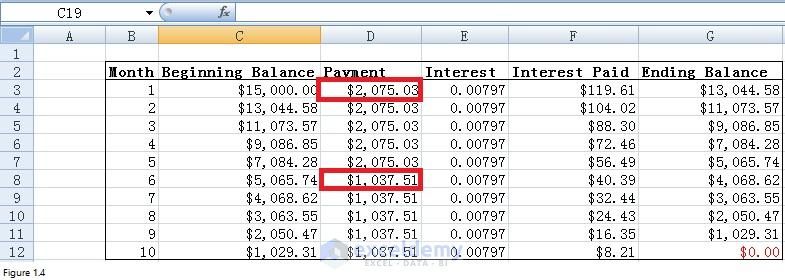Figure 1.4

## Case 2: Calculate the least amount of money that you need to save in the first year for your retiree plan

Suppose that you will deposit some money into your retirement account at beginning of this year and each of the next 39 years. Each year, you plan to increase your retirement contribution by \$500. When you retire 40 years later, you plan to withdraw \$100,000 at beginning of each year for 20 years. Assume that the investments will earn 10 percent per year during the first 20 years and 5 percent per year during all other years, what is the least amount of money you can deposit this year and still have enough to make your retirement withdrawal?

To set up the model, I made a worksheet like that in Figure 2.1. The amount of money that you need to contribute to your retirement account each year will be entered into column D. And the amount of money that you withdrawal each year will be entered into column F. Column E gives you the return percent for your investment each year. Column C and column G tell you how much money in your account at the beginning or the end of each year respectively. The relationship between these columns can be described by the following equation:

(Initial Balance + Deposit)*Return Percent – Withdrawal = Ending Balance

And the formula “=(C3+D3-F3)*(1+E3)” which is derived from this equation was put in cell G3 to compute the ending balance in the first year. By copying this formula from G3 into range G4:G62, we can get the ending balance for other years. Initial balance of this year should equal to the ending balance of last year. Therefore I put formula “=G3” in cell C4 to get an initial balance for the second year. This formula was copied into cells C5 through C62 to get the initial balance for other years.

From the problem, we can know that cell D3 is our target cell as what we need to know is exactly how much we need to save in the first year and thus I will leave it blank at the moment. Interesting, cell D3 is also our by changing cell. Please note that target cell and by changing cell is the same in our case. Since you need to increase your investment by \$500 each year, formula “=D3+500” was entered into cell D4 to calculate how much you need to deposit in the second year. Then copied this formula from cell D4 to D5:D42 to make sure that the retirement contribution increases by \$500 per year during Years 3 through 40.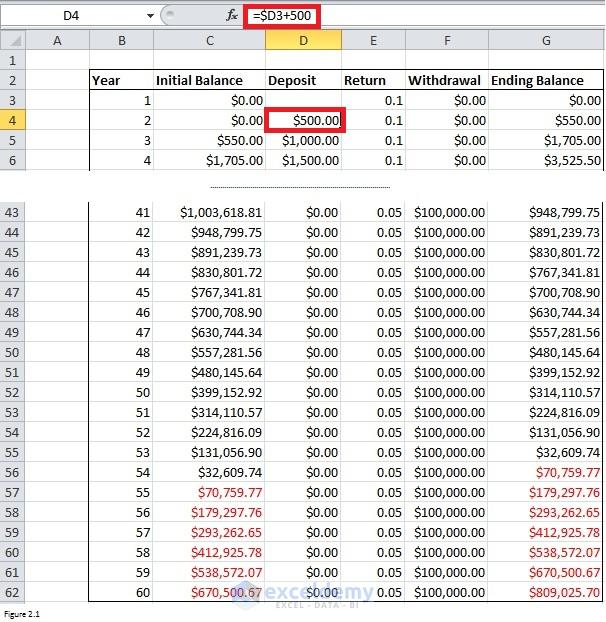Figure 2.1

Click on Solver in Analysis group to open Solver Parameters dialog box. The solver Parameter dialog box for this model is shown in Figure 2.2. The constraint for this model is “\$G\$43:\$G\$62>=0” because you need to make sure that the ending balance for years 41 through 60 is nonnegative. This model is linear because the constraint can be described in a linear relationship.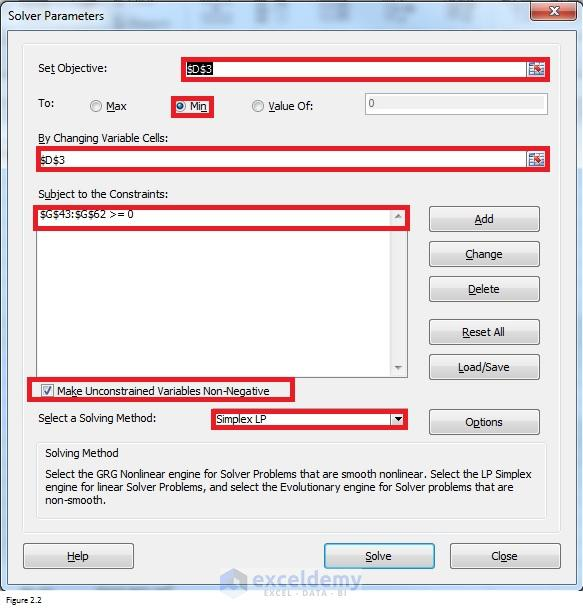Figure 2.2

After clicking Solve in the Solver Parameters dialog box, you can find that you should save at least \$1,569.41 into your account in the first year.

1. Reply